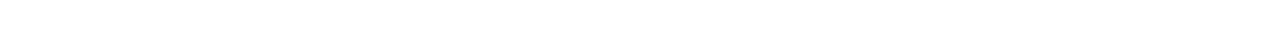# 17.2.4 Natural Selection: Hardy-Weinberg Principle

### Natural Selection: Hardy-Weinberg Principle

• The Hardy-Weinberg principle states that if certain conditions are met then the allele frequencies of a gene within a population will not change from one generation to the next
• There are seven conditions or assumptions that must be met for the Hardy-Weinberg principle to hold true
• The Hardy-Weinberg equation allows for the calculation of allele and genotype frequencies within populations
• It also allows for predictions to be made about how these frequencies will change in future generations

#### Conditions for the Hardy-Weinberg principle

• Organisms are diploid
• Organisms reproduce by sexual reproduction only
• There is no overlap between generations
• Mating is random
• The population is infinitely large
• There is no migration, mutation or selection
• Allele frequencies are equal in both sexes

#### Hardy-Weinberg equations

• If the phenotype of a trait in a population is determined by a single gene with only two alleles (we will use B / b as examples throughout this section), then the population will consist of individuals with three possible genotypes:
• Homozygous dominant (BB)
• Heterozygous (Bb)
• Homozygous recessive (bb)
• When using the Hardy-Weinberg equations the frequency of a genotype is represented as a proportion of the population
• For example, the BB genotype could be 0.40
• Whole population = 1
• The letter p represents the frequency of the dominant allele (B)
• The letter q represents the frequency of the recessive allele (b)
• As there are only two alleles at a single gene locus for this phenotypic trait in the population:

p + q = 1

• The chance of an individual being homozygous dominant is p2
• In this instance, the offspring would inherit dominant alleles from both parents ( p x p = p2 )
• The chance of an individual being heterozygous is 2pq
• Offspring could inherit a dominant allele from the father and a recessive allele from the mother ( p x q ) or offspring could inherit a dominant allele from the mother and a recessive allele from the father ( p x q ) = 2pq
• The chance of an individual being homozygous recessive is q2
• In this instance, the offspring would inherit recessive alleles from both parents ( q x q = q2 )
• As these are all the possible genotypes of individuals in the population the following equation can be constructed:

p2 + q2 + 2pq = 1

#### Worked example: Calculating frequencies of genotypes

In a population of birds, 10% of the individuals exhibit the recessive phenotype of white feathers.

Calculate the frequencies of all genotypes.

Solution:

• We will use F / f to represent dominant and recessive alleles for feather colour
• Those with the recessive phenotype must have the homozygous recessive genotype, ff
• Therefore q2 = 0.10 (as 10% of the individuals have the recessive phenotype and q2 represents this)

To calculate the frequencies of the homozygous dominant ( p2 ) and heterozygous ( 2pq ):

• Step 1:  Find q:• Step 2:  Find p (the frequency of the dominant allele F). If q = 0.32, and p + q = 1 then:

p + q = 1

p = 1 – 0.32

p = 0.68

• Step 3:  Find p2 (the frequency of homozygous dominant genotype):

0.682 = 0.46

p2 = 0.46

• Step 4:  Find 2pq = 2 x (p) x (q):

2 x (0.68) x (0.32) = 0.44

• Step 5:  Check calculations by substituting the values for the three frequencies into the equation; they should add up to 1:

p2 + 2pq + q2 = 1

0.46 + 0.44 + 0.10 = 1

In summary:

• Allele frequencies:
• p = F = 0.68
• q = f = 0.32
• Genotype frequencies:
• p2 = FF = 0.46
• q2 = ff = 0.10
• 2pq = Ff = 0.44

#### Exam Tip

When you are using Hardy-Weinberg equations, start your calculations determining the proportion of individuals that display the recessive phenotype – you will always know the genotype for this: homozygous recessive. Remember that the dominant phenotype is seen in both homozygous dominant, and heterozygous individuals.

Also, don’t mix up the Hardy-Weinberg equations with the Hardy-Weinberg principle. The equations are used to estimate the allele and genotype frequencies in a population. The principle suggests that there is an equilibrium between allele frequencies and there is no change in this between generations.### Author: Lára

Lára graduated from Oxford University in Biological Sciences and has now been a science tutor working in the UK for several years. Lára has a particular interest in the area of infectious disease and epidemiology, and enjoys creating original educational materials that develop confidence and facilitate learning.
CloseClose